# HBSE 10th Class Maths Solutions Chapter 6 Triangles Ex 6.3

Haryana State Board HBSE 10th Class Maths Solutions Chapter 6 Triangles Ex 6.3 Textbook Exercise Questions and Answers.

## Haryana Board 10th Class Maths Solutions Chapter 6 Triangles Ex 6.3

Question 1.
State which pairs of triangles in below figures are similar. Write the similarity criterion used by you for answering the question and also write the pairs of similar triangles in the symbolic form :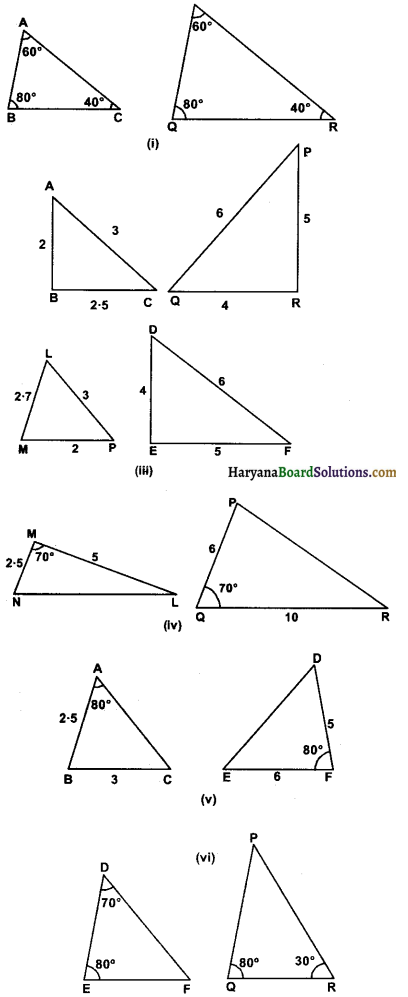Solution:
(i) In ∆ABC and ∆PQR
∠A = ∠P = 60°
∠B = ∠Q = 80°
∠C = ∠R = 40°
By AAA similarity criterion.
∆ABC ~ ∆PQR.

(ii) In ∆ABC and ∆QRP
$$\frac{\mathrm{AB}}{\mathrm{QR}}=\frac{2}{4}=\frac{1}{2}$$
$$\frac{\mathrm{BC}}{\mathrm{RP}}=\frac{2 \cdot 5}{5}=\frac{1}{2}$$
$$\frac{\mathrm{AC}}{\mathrm{PQ}}=\frac{3}{6}=\frac{1}{2}$$
∵ $$\frac{\mathrm{AB}}{\mathrm{QR}}=\frac{\mathrm{BC}}{\mathrm{RP}}=\frac{\mathrm{AC}}{\mathrm{PQ}}$$
∴ By SSS similarity criterion
∆ABC ~ ∆QRP

(iii) In ∆LMP and ∆DEF
$$\frac{\mathrm{LM}}{\mathrm{DE}}=\frac{2 \cdot 7}{4}$$ = 0.675
$$\frac{\mathrm{MP}}{\mathrm{EF}}=\frac{2}{5}$$ = 0.4
$$\frac{\mathrm{LP}}{\mathrm{DF}}=\frac{3}{6}$$ = 0.5
∵ $$\frac{\mathrm{LM}}{\mathrm{DF}} \neq \frac{\mathrm{MP}}{\mathrm{EF}} \neq \frac{\mathrm{LP}}{\mathrm{DF}}$$
∴ These two triangles are not similar because they do not satisfy the SSS similarity criterion.(iv) In ∆MNL and ∆QPR
∠M = ∠Q = 70°
$$\frac{\mathrm{MN}}{\mathrm{PQ}}=\frac{2 \cdot 5}{6}$$ (approx)
$$\frac{\mathrm{ML}}{\mathrm{QR}}=\frac{5}{10}$$ = 0.5
∵ $$\frac{\mathrm{MN}}{\mathrm{PQ}} \neq \frac{\mathrm{ML}}{\mathrm{QR}}$$
∴ These two triangles are not similar because they do not satisfy the SAS similarity criterion.

(v) In ∆ABC and ∆FDE
∠A = ∠F = 80°
$$\frac{\mathrm{AB}}{\mathrm{DF}} \neq \frac{\mathrm{AC}}{\mathrm{EF}}$$ [∵ AC is not given]
∴ These two triangles are not similar because they do not satisfy the SAS similarity criterion.

(vi) In ∆DEF and ∆PQR
∠P = 180° (80° + 30°)
∠P = 180° – 110°
∠P = 70°
Now, ∠D = ∠P = 70°
∠E = ∠Q = 80°
By AA similarity criterion ∆DEF ~ ∆PQR.Question 2.
In given figure ∆ODC – ∆OBA, ∠BOC = 125° and ∠CDO = 70°. Find ∠DOC, ∠DCO and ∠OAB.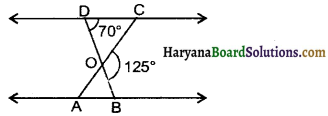Solution:
We have, ∠CDO = 70° and ∠COB = 125°
∵ BD is a line and CO ray on it
∠DOC + ∠COB = 180°
∠DOC + 125° = 1800
∠DOC = 180° – 125°
∠DOC = 55°
In ∆COD, we have
∠DOC + ∠DCO + ∠CDO = 180° [Sum of ∠s of a triangle is 180°]
55° + ∠DCO + 70° = 180°
125° + ∠DCO = 180°
∠DCO = 180° – 125°
∠DCO = 55°
Now ∆ODC ~ ∆OBA (given)
∴ ∠OAB = ∠DCO
(Corresponding ∠s of similar ∆s)
∠OAB = 55°
Hence, ∠DOC = 55°, ∠DCO = 55°, ∠OAB = 55°.Question 3.
Diagonals AC and BD of a trapezium ABCD with AB DC ntersect each other at the point O. Using a similarity criterion for two triangles, show that $$\frac{\mathrm{OA}}{\mathrm{OC}}=\frac{\mathrm{OB}}{\mathrm{OD}}$$.
Solution:
Given : ABCD is a trapezium in which AB || DC. Its diagonals AC and BD meet at O.
To Prove: $$\frac{\mathrm{OA}}{\mathrm{OC}}=\frac{\mathrm{OB}}{\mathrm{OD}}$$
∠OAB = ∠OCD [∵ AB || DC]
∴ Alternate ∠s are equal.
∠AOB = ∠COD (vertically opposite ∠s)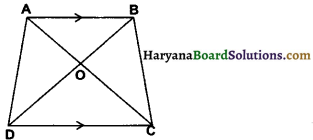∴ ∆OAB ~ ∆OCD (By AA similarity criterion)
∴ $$\frac{\mathrm{OA}}{\mathrm{OC}}=\frac{\mathrm{OB}}{\mathrm{OD}}$$
Hence Proved.

Question 4.
In given figure $$\frac{\mathrm{QR}}{\mathrm{QS}}=\frac{\mathrm{QT}}{\mathrm{PR}}$$ and ∠1 = ∠2. Show that ∆PQS ~ ∆TQR.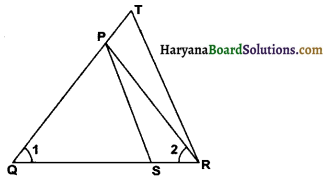Solution:
Given: $$\frac{\mathrm{QR}}{\mathrm{QS}}=\frac{\mathrm{QT}}{\mathrm{PR}}$$ and ∠1 = ∠2.
To Prove: ∆PQS ~ ∆TQR
Proof: We have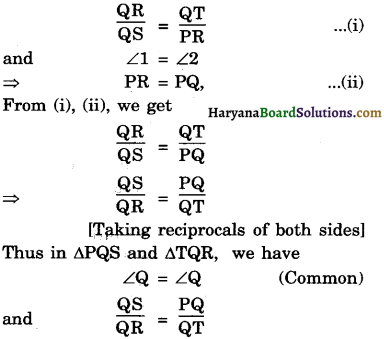∴ ∆PQS ~ ∆TQR [By SAS similarity criterion]
Hence Proved.Question 5.
S and T are points on sides PR and QR of ∆PQR such that ∠P = ∠RTS. Show that ∆RPQ – ∆RTS.
Solution :
Given : S and T are points on sides PR and QR of ∆PQR such that ∠P = ∠RTS
To Prove: ∆RPQ ~ ∆RTS.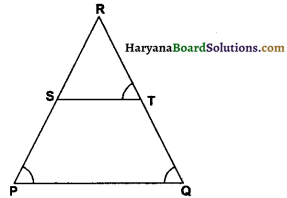Proof: In ∆RPQ and ∆RTS.
∠P = ∠RTS (given)
∠R = ∠R (common)
∴ ∆RPQ ~ ∆RTS (By AA similarity criterion)
Hence Proved.

Question 6.
In given figure, If ∆ABE ≅ ∆ACD, show that ∆ADE ~ ∆ABC.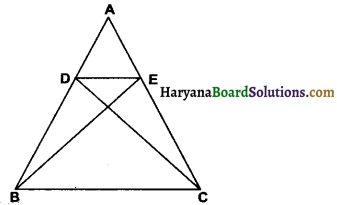Solution:
Given: ∆ABE ≅ ∆ACD
Proof: ∆ABE ~ ∆ACD
∴ AB = AC
⇒ $$\frac{\mathrm{AB}}{\mathrm{AC}}$$ = 1 and $$\frac{\mathrm{AD}}{\mathrm{AE}}$$ = 1
⇒ $$\frac{\mathrm{AB}}{\mathrm{AC}}=\frac{\mathrm{AD}}{\mathrm{AE}}$$
Now, In ∆ADE and ∆ABC, we have
⇒ $$\frac{\mathrm{AB}}{\mathrm{AC}}=\frac{\mathrm{AD}}{\mathrm{AE}}$$
⇒ $$\frac{\mathrm{AB}}{\mathrm{AD}}=\frac{\mathrm{AC}}{\mathrm{AE}}$$
and ∠DAE = ∠BAC (each = ∠A)
∆ADE ~∆ABC. [By AA similarity criterion]
Hence Proved .Question 7.
In given figure altitudes AD and CE of AABC intersect each other at the point P. Show that :
(i) ∆AEP ~ ∆CDP
(ii) ∆ABD ~ ∆CBE
(iv) ∆PDC ~ ∆BEC.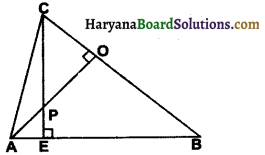Solution :
Given : CE ⊥ AB and AD ⊥ BC.
To Prove : (i) ∆AEP ~ ∆CDP
(ii) ∆ABD ~ ∆CBE
(iv) ∆PDC ~ ∆BEC.
Proof : (i) In ∆AEP and ∆CDP
∠AEP = ∠CDP (each = 90°)
∠APE = ∠CPD (vertically opposite ∠s)
∴ ∆AEP ~ ∆CDP
(By AA similarity criterion) Hence Proved.

(ii) In ∆ABD and ∆CBE
∠B = ∠B (common)
∴ ∆ABD ~ ∆CBE
(By AA similarity criterion) Hence Proved.

∠AEP = ∠ADB (each = 90°)
∠PAE = ∠DAB (same angle)
(By AA similarity criterion) Hence Proved.

(iv) In ∆PDC and ∆BEC
∠PDC = ∠BEC (each = 90°)
∠PCD = ∠BCE (same angle)
∆PDC ~ ∆BEC
(By AA similarity criterion) Hence Proved.Question 8.
E is a point on the side AD produced of a parallelogram ABCD and BE intersects CD at F. Show that ∆ABE ~ ∆CFB.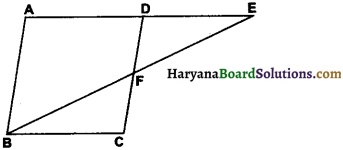Solution :
Given : E is a point on side AD produced of a ||gm ABCD and BE intersects CD at F.
To Prove : ∆ABE ~ ∆CFB.
Proof : In ∆ABE and ∆CFB.
∠AEB = ∠CBF (alternate interior ∠s)
∠A = ∠C (opposite ∠s of a ||gm )
∴ ∆ABE ~ ∆CFB
(By AA similarity criterion) Hence Proved.

Question 9.
In given figure, ABC and AMP are two right triangles, right angled at B and M respectively. Prove that :
(i) ∆ABC ~ ∆AMP.
(ii) $$\frac{C A}{P A}=\frac{B C}{M P}$$.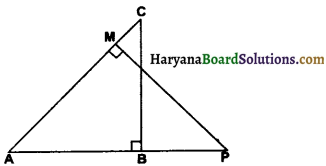Solution:
Given: ∆ABC and ∆AMP are two right angled triangles right angles at B and M respectively.
To Prove :(i) ∆ABC ~ ∆AMP.
(ii) $$\frac{C A}{P A}=\frac{B C}{M P}$$.
Proof : (i) In ∆ABC and ∆AMP.
∠ABC = ∠AMP (each = 90°)
∠A = ∠A (common)
∴ ∆ABC ~ ∆AMP (By AA similarity criterion)
Hence Proved.

(ii) ∵ ∆ABC ~ ∆AMP
∴ $$\frac{\mathrm{CA}}{\mathrm{PA}}=\frac{\mathrm{BC}}{\mathrm{MP}}$$
(corresponding sides of similar triangles)
Hence Proved.Question 10.
CD and GH are respectively the bisectors of ∠ACB and ∠EGF such that D and H lie on sides AB and FE of ∆ABC and ∆EFG respectively. If ∆ABC ~ ∆FEG, show that:
(i) $$\frac{\mathrm{CD}}{\mathrm{GH}}=\frac{\mathrm{AC}}{\mathrm{FG}}$$
(ii) ∆DCB ~ ∆HGE
(iii) ∆DCA ~ ∆HGF
Solution:
Given : CD and GH are respectively the bisectors of ∠ACB and ∠EGF such that D and H lie on the sides AB and EF of ∆ABC and ∆EFG and ∆ABC ~ ∆FEG.
To Prove :
(i) ∆DCB ~ ∆HGE
(ii) ∆DCA ~ ∆HGF
(iii) $$\frac{\mathrm{CD}}{\mathrm{GH}}=\frac{\mathrm{AC}}{\mathrm{FG}}$$
Proof : (i) ∆ABC ~ ∆FEG (given)
∠ACB = ∠EGF (corresponding ∠s of similar ∆s)
$$\frac{1}{2}$$ ∠ACB = $$\frac{1}{2}$$ ∠EGF
[CD and GH are bisectors of ∠C and ∠G.]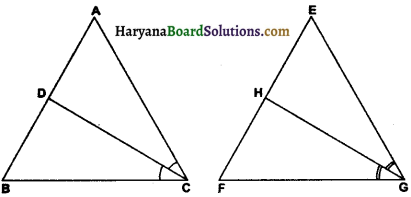∠DCB = ∠HGE ………………..(1)
and ∠B = ∠E
(corresponding ∠s of similar ∆s)
⇒ ∠DBC = ∠HEG ……………….(2)
From (1) and (2), we have
∆DCB ~ ∆HGE
Hence Proved.

(ii) In ∆DCA and ∆HGF
∠C = ∠G
(corresponding ∠s of similar ∆s)
∠ACD = ∠HGF [CD and GH are bisectors of ∠C and ∠G] ………………..(1)
∠A = ∠F
(corresponding ∠s of similar ∆s) ……………….(2)
(third ∠s of two triangles) …………………(3)
∴ ∆DCA ~ ∆HGF. (By AAA similarity criterion)
Hence Proved.

(iii) ∵ ∆DCA ~ ∆HGF.
∴ $$\frac{\mathrm{CD}}{\mathrm{GH}}=\frac{\mathrm{AC}}{\mathrm{FG}}$$
(corresponding sides of similar ∆s)
Hence Proved.Question 11.
In given figure, E is a point on side CB produced of an isosceles triangle ABC with AB = AC If AD ⊥ BC and EF ⊥ AC, prove that ∆ABD ~ ∆ECF.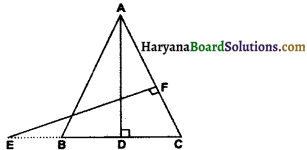Solution :
Given : AB = AC, AD ⊥ BC and EF ⊥ AC.
To Prove : ∆ABD ~ ∆ECF
Proof : AB = AC (given)
∠B = ∠C [∵ ∠s opposite to equal side are equal]
Now, In ∆ABD and ∆ECF,
∠ABD = ∠ECF [∵ ∠B = ∠C]
[∵ AD ⊥ BC and EF ⊥ AC]
∴ ∠ABD ~ ∆ECF
(By AA similarity criterion)
Hence Proved.

Question 12.
Sides AB and BC and median AD of a triangle ABC are respectively proportional to sides PQ and QR and median PM of ∆PQR (see figure below). Show that ∆ABC ~ ∆PQR.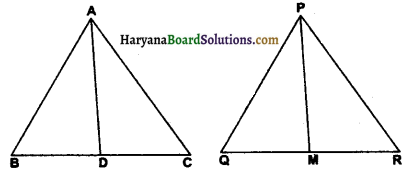Solution: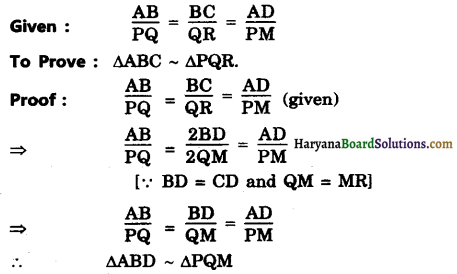(By SSS similarity criterion)
∠B = ∠Q
(Corresponding ∠s of Similar As)
Now, in ∆ABC and ∆PQR.
$$\frac{\mathrm{AB}}{\mathrm{PQ}}=\frac{\mathrm{BC}}{\mathrm{QR}}$$ (given)
∠B = ∠Q (Proved above)
∴ ∆ABC ~ ∆PQR [By SAS similarity criterion]
Hence Proved.Question 13.
D is a point on the side BC of a trianlge ABC such that ∠ADC = ∠BAC. Show that CA2 = CB.CD
Solution:
To prove : CA2 = CB.CD
Proof : In ∆ABC and ∆DAC
∠C = ∠C (common)
∆ABC ~ ∆DAC (By AA similarity criterion)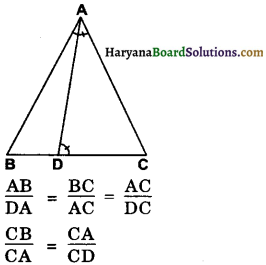⇒ CA2 = CB × CD.
Hence Proved.

Question 14.
Sides AB and AC and median AD of a triangle ABC are respectively proportional to sides PQ and PR and median PM of another triangle PQR. Show that ∆ABC ~ ∆PQR.
Solution :
Given : In ∆ABC and ∆PQR
$$\frac{\mathrm{AB}}{\mathrm{PQ}}=\frac{\mathrm{AC}}{\mathrm{PR}}=\frac{\mathrm{AD}}{\mathrm{PM}}$$
To Prove : ∆ABC ~ ∆PQR
Construction : Produce AD to E such that AD = DE and PM to N such that PM = MN Join CE and NR.
Proof : In ∆ADB and ∆EDC
BD = CD (AD is median)
∠ADB = ∠CDE (vertically opposite ∠s)
∆ADB ≅ ∆EDC (By SAS Congruence criterion)
⇒ AB = EC (By CPCT) …………….(1)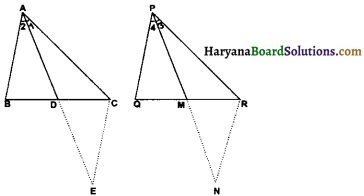Similarly, ∆PMQ ≅ ∆NMR (By SAS congruence criterion)
⇒ PQ = NR (By CPCT) …………..(2)
Now, $$\frac{\mathrm{AB}}{\mathrm{PQ}}=\frac{\mathrm{AC}}{\mathrm{PR}}=\frac{\mathrm{AD}}{\mathrm{PM}}$$(given)
⇒ $$\frac{\mathrm{EC}}{\mathrm{NR}}=\frac{\mathrm{AC}}{\mathrm{PR}}=\frac{\mathrm{AD}}{\mathrm{PM}}$$
[From (1) and (2), AB = EC and PQ = NR]
⇒ $$\frac{\mathrm{EC}}{\mathrm{NR}}=\frac{\mathrm{AC}}{\mathrm{PR}}=\frac{2 \mathrm{AD}}{2 \mathrm{PM}}$$
⇒ $$\frac{\mathrm{EC}}{\mathrm{NR}}=\frac{\mathrm{AC}}{\mathrm{PR}}=\frac{\mathrm{AE}}{\mathrm{PN}}$$
[By construction AD = DE and PM = MN)
∴ ∆ACE ~ ∆PRN (By SSS similarity criterion)
∠1 = ∠3 ……………….(3)
(Corresponding ∠s of similar triangles)
Similarly ∠2 = ∠4 ………………(4)
Adding (3) and (4) we get
∠1 + ∠2 = ∠3 + ∠4.
⇒ ∠A = ∠P.
Now ∆ABC and ∆PQR.
∠A = ∠P (Proved above)
$$\frac{\mathrm{AB}}{\mathrm{PQ}}=\frac{\mathrm{AC}}{\mathrm{PR}}$$ (given)
∴ ∆ABC ~ ∆PQR.Question 15.
A vertical pole of length 6m casts a shadow 4m long on the ground and at the same time a tower casts a shadow 28m long. Find the height of the tower.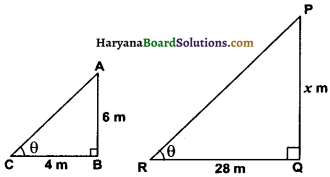Solution:
Let AB be the vertical pole of length 6m casts a shadow BC and PQ be the tower casts a shadow QR. Let height of tower berm. Join AC and PR.
In ∆ABC and ∆PQR
∠B = ∠Q (each = 90°)
∠C = ∠R (Angle of elevation of the Sun)
∴ ∆ABC ~ ∆PQR (By AA similarity criterion)
∴ $$\frac{\mathrm{AB}}{\mathrm{PQ}}=\frac{\mathrm{BC}}{\mathrm{QR}}$$
[Corresponding sides of similar triangles]
⇒ $$\frac{6}{x}=\frac{4}{28}$$
⇒ x = $$\frac{6 \times 28}{4}$$
x = 42.
Hence, height of tower = 42 m.Question 16.
If AD and PM are medians of triangles ABC and PQR, respectively where ∆ABC ~ ∆PQR, prove that $$\frac{\mathrm{AB}}{\mathrm{PQ}}=\frac{\mathrm{AD}}{\mathrm{PM}}$$.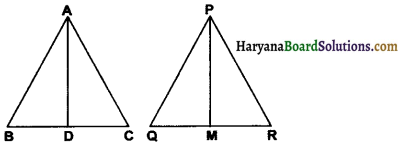Solution :
Given : AD and PM are medians of ∆ABC and ∆PQR respectively and ∆ABC ~ ∆PQR.
To Prove: $$\frac{\mathrm{AB}}{\mathrm{PQ}}=\frac{\mathrm{AD}}{\mathrm{PM}}$$
Proof : ∆ABC ~ ∆PQR
∴ $$\frac{\mathrm{AB}}{\mathrm{PQ}}=\frac{\mathrm{AC}}{\mathrm{PR}}=\frac{\mathrm{BC}}{\mathrm{QR}}$$ ………………(1)
(corresponding sides of similar triangles)
⇒ $$\frac{\mathrm{AB}}{\mathrm{PQ}}=\frac{\mathrm{BC}}{\mathrm{QR}}$$
⇒ $$\frac{\mathrm{AB}}{\mathrm{PQ}}=\frac{2 \mathrm{BD}}{2 \mathrm{QM}}$$
[∵ AD and PM are medians
∴ BD = CD and QM = MR]
⇒ $$\frac{\mathrm{AB}}{\mathrm{PQ}}=\frac{\mathrm{BD}}{\mathrm{QM}}$$
and ∠B = ∠Q
[Corresponding ∠s of similar ∆s ABC and PQR]
∴ ∆ADB ~ ∆PMQ [By SAS similarity criterion]
$$\frac{\mathrm{AB}}{\mathrm{PQ}}=\frac{\mathrm{AD}}{\mathrm{PM}}$$ (Corresponding sides of similar ∆s.)
Hence Proved.